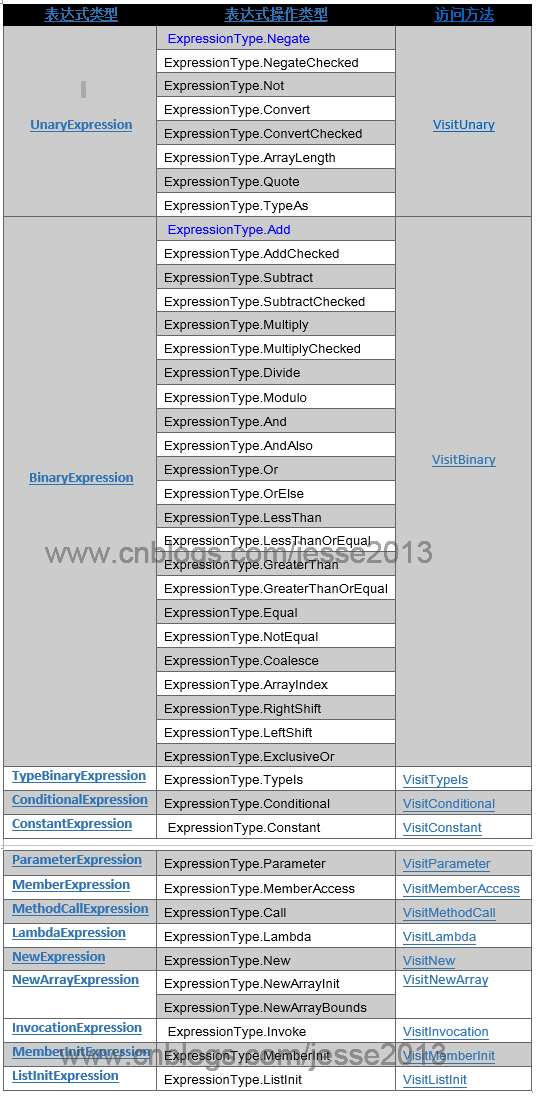# 由浅入深表达式树（二）遍历表达式树 - 腾飞（Jesse) - 博客园为什么要学习表达式树？表达式树是将我们原来可以直接由代码编写的逻辑以表达式的方式存储在树状的结构里，从而可以在运行时去解析这个树，然后执行，实现动态的编辑和执行代码。LINQ to SQL就是通过把表达式树翻译成SQL来实现的，所以了解表达树有助于我们更好的理解 LINQ to SQL，同时如果你有兴趣，可以用它创造出很多有意思的东西来。

# 有返回值的表达式树

 1 2 3 4 5 6 7 8 9 10 11 12 13 14 15 16 17 18 19 20 21 22 23 24 25 26 27 28 29 30 31 32 33 34 35 36 `// 直接返回常量值` `ConstantExpression ce1 = Expression.Constant(10);` `            ` `// 直接用我们上面创建的常量表达式来创建表达式树` `Expression> expr1 = Expression.Lambda>(ce1);` `Console.WriteLine(expr1.Compile().Invoke());` `// 10` `// --------------在方法体内创建变量，经过操作之后再返回------------------` `// 1.创建方法体表达式 2.在方法体内声明变量并附值 3. 返回该变量` `ParameterExpression param2 = Expression.Parameter(``typeof``(``int``));` `BlockExpression block2 = Expression.Block(` `    ``new``[]{param2},` `    ``Expression.AddAssign(param2,Expression.Constant(20)),` `    ``param2` `    ``);` `Expression> expr2 = Expression.Lambda>(block2);` `Console.WriteLine(expr2.Compile().Invoke());` `// 20` `// -------------利用GotoExpression返回值-----------------------------------` `LabelTarget returnTarget = Expression.Label(``typeof``(Int32));` `LabelExpression returnLabel = Expression.Label(returnTarget,Expression.Constant(10,``typeof``(Int32)));` `// 为输入参加+10之后返回` `ParameterExpression inParam3=Expression.Parameter(``typeof``(``int``));` `BlockExpression block3 = Expression.Block(` `    ``Expression.AddAssign(inParam3,Expression.Constant(10)),` `    ``Expression.Return(returnTarget,inParam3),` `    ``returnLabel);` `Expression> expr3 = Expression.Lambda>(block3,inParam3);` `Console.WriteLine(expr3.Compile().Invoke(20));` `// 30`

 1 2 3 4 5 6 7 8 9 10 11 12 13 14 `//简单的switch case 语句` `ParameterExpression genderParam = Expression.Parameter(``typeof``(``int``));` `SwitchExpression swithExpression = Expression.Switch(` `    ``genderParam,` `    ``Expression.Constant(``"不详"``), ``//默认值` `    ``Expression.SwitchCase(Expression.Constant(``"男"``),Expression.Constant(1)), ` `Expression.SwitchCase(Expression.Constant(``"女"``),Expression.Constant(0))` `//你可以将上面的Expression.Constant替换成其它复杂的表达式,ParameterExpression, BinaryExpression等, 这也是表达式灵活的地方, 因为归根结底它们都是继承自Expression, 而基本上我们用到的地方都是以基类作为参数类型接受的,所以我们可以传递任意类型的表达式。` `    ``);` `Expression> expr4 = Expression.Lambda>(swithExpression, genderParam);` `Console.WriteLine(expr4.Compile().Invoke(1)); ``//男` `Console.WriteLine(expr4.Compile().Invoke(0)); ``//女` `Console.WriteLine(expr4.Compile().Invoke(11)); ``//不详`

# 表达式的遍历1 2 3 4 5 6 7 8 9 `List myUsers = ``new` `List();` `var` `userSql = myUsers.AsQueryable().Where(u => u.Age > 2);` `Console.WriteLine(userSql);` `// SELECT * FROM (SELECT * FROM User) AS T WHERE (Age>2)` `List myUsers2 = ``new` `List();` `var` `userSql2 = myUsers.AsQueryable().Where(u => u.Name==``"Jesse"``);` `Console.WriteLine(userSql2);` `// SELECT * FROM (SELECT * FROM USER) AS T WHERE (Name='Jesse')`

 1 2 3 4 5 6 7 8 9 10 11 12 13 14 15 16 17 18 19 `public` `static` `class` `Queryable` `{` `    ``public` `static` `IQueryable Where(``this` `IQueryable source, Expression> predicate)` `    ``{` `        ``if` `(source == ``null``)` `        ``{` `            ``throw` `new` `ArgumentNullException(``"source"``);` `        ``}` `        ``if` `(predicate == ``null``)` `        ``{` `            ``throw` `new` `ArgumentNullException(``"predicate"``);` `        ``}` `        ``return` `source.Provider.CreateQuery(` `            ``Expression.Call(``null``, ((MethodInfo)MethodBase.GetCurrentMethod())` `            ``.MakeGenericMethod(``new` `Type[] { ``typeof``(TSource) }),` `            ``new` `Expression[] { source.Expression, Expression.Quote(predicate) }));` `    ``}` `}`

 1 2 3 4 5 6 7 8 9 10 11 12 13 `public` `static` `class` `QueryExtensions` `{` `    ``public` `static` `string` `Where(``this` `IQueryable source,` `        ``Expression> predicate)` `    ``{` `        ``var` `expression = Expression.Call(``null``, ((MethodInfo)MethodBase.GetCurrentMethod())` `        ``.MakeGenericMethod(``new` `Type[] { ``typeof``(TSource) }),` `        ``new` `Expression[] { source.Expression, Expression.Quote(predicate) });` `        ``var` `translator = ``new` `QueryTranslator();` `        ``return` `translator.Translate(expression);` `    ``}` `}`

 1 2 3 4 5 6 7 8 9 `class` `QueryTranslator : ExpressionVisitor` `{` `    ``internal` `string` `Translate(Expression expression)` `    ``{` `        ``this``.sb = ``new` `StringBuilder();` `        ``this``.Visit(expression);` `        ``return` `this``.sb.ToString();` `    ``}` `}`

 1 2 3 4 5 6 7 8 9 10 11 12 13 `protected` `override` `Expression VisitMethodCall(MethodCallExpression m)` `{` `    ``if` `(m.Method.DeclaringType == ``typeof``(QueryExtensions) && m.Method.Name == ``"Where"``)` `    ``{` `        ``sb.Append(``"SELECT * FROM ("``);` `        ``this``.Visit(m.Arguments);` `        ``sb.Append(``") AS T WHERE "``);` `        ``LambdaExpression lambda = (LambdaExpression)StripQuotes(m.Arguments);` `        ``this``.Visit(lambda.Body);` `        ``return` `m;` `    ``}` `    ``throw` `new` `NotSupportedException(``string``.Format(``"方法{0}不支持"``, m.Method.Name));` `}`

 1 2 3 4 5 6 7 8 9 10 11 12 13 14 15 16 17 18 19 20 21 22 23 24 25 26 27 28 29 30 31 32 33 34 35 36 37 `protected` `override` `Expression VisitBinary(BinaryExpression b)` `{` `    ``sb.Append(``"("``);` `    ``this``.Visit(b.Left);` `    ``switch` `(b.NodeType)` `    ``{` `        ``case` `ExpressionType.And:` `            ``sb.Append(``" AND "``);` `            ``break``;` `        ``case` `ExpressionType.Or:` `            ``sb.Append(``" OR"``);` `            ``break``;` `        ``case` `ExpressionType.Equal:` `            ``sb.Append(``" = "``);` `            ``break``;` `        ``case` `ExpressionType.NotEqual:` `            ``sb.Append(``" <> "``);` `            ``break``;` `        ``case` `ExpressionType.LessThan:` `            ``sb.Append(``" < "``);` `            ``break``;` `        ``case` `ExpressionType.LessThanOrEqual:` `            ``sb.Append(``" <= "``);` `            ``break``;` `        ``case` `ExpressionType.GreaterThan:` `            ``sb.Append(``" > "``);` `            ``break``;` `        ``case` `ExpressionType.GreaterThanOrEqual:` `            ``sb.Append(``" >= "``);` `            ``break``;` `        ``default``:` `            ``throw` `new` `NotSupportedException(``string``.Format(“二元运算符{0}不支持”, b.NodeType));` `    ``}` `    ``this``.Visit(b.Right);` `    ``sb.Append(``")"``);` `    ``return` `b;` `}`

 1 2 3 4 5 6 7 8 9 10 11 12 13 14 15 16 17 18 19 20 21 22 23 24 25 26 27 28 29 30 31 32 33 34 35 36 37 38 39 40 41 42 43 44 `protected` `override` `Expression VisitConstant(ConstantExpression c)` `{` `    ``IQueryable q = c.Value ``as` `IQueryable;` `    ``if` `(q != ``null``)` `    ``{` `        ``// 我们假设我们那个Queryable就是对应的表` `        ``sb.Append(``"SELECT * FROM "``);` `        ``sb.Append(q.ElementType.Name);` `    ``}` `    ``else` `if` `(c.Value == ``null``)` `    ``{` `        ``sb.Append(``"NULL"``);` `    ``}` `    ``else` `    ``{` `        ``switch` `(Type.GetTypeCode(c.Value.GetType()))` `        ``{` `            ``case` `TypeCode.Boolean:` `                ``sb.Append(((``bool``)c.Value) ? 1 : 0);` `                ``break``;` `            ``case` `TypeCode.String:` `                ``sb.Append(``"'"``);` `                ``sb.Append(c.Value);` `                ``sb.Append(``"'"``);` `                ``break``;` `            ``case` `TypeCode.Object:` `                ``throw` `new` `NotSupportedException(``string``.Format(``"The constant for '{0}' is not supported"``, c.Value));` `            ``default``:` `                ``sb.Append(c.Value);` `                ``break``;` `        ``}` `    ``}` `    ``return` `c;` `}` `protected` `override` `Expression VisitMember(MemberExpression m)` `{` `    ``if` `(m.Expression != ``null` `&& m.Expression.NodeType == ExpressionType.Parameter)` `    ``{` `        ``sb.Append(m.Member.Name);` `        ``return` `m;` `    ``}` `    ``throw` `new` `NotSupportedException(``string``.Format(``"The member '{0}' is not supported"``, m.Member.Name));` `}`

1. 重写IQuerable的Where方法，构造MethodCallExpression传给我们的表达式访问类。
2. 在我们的表达式访问类中重写相应的具体访问方法。
3. 在具体访问方法中，解释表达式，翻译成SQL语句。

http://msdn.microsoft.com/en-us/library/bb397951(v=vs.120).aspx
http://msdn.microsoft.com/en-us/library/system.linq.expressions.aspx
http://msdn.microsoft.com/en-us/library/system.linq.expressions.expression.aspx
http://blogs.msdn.com/b/mattwar/archive/2007/07/30/linq-building-an-iqueryable-provider-part-i.aspx

### 觉得文章有用就打赏一下文章作者

#### 支付宝扫一扫打赏#### 微信扫一扫打赏• QQ咨询
• 回顶